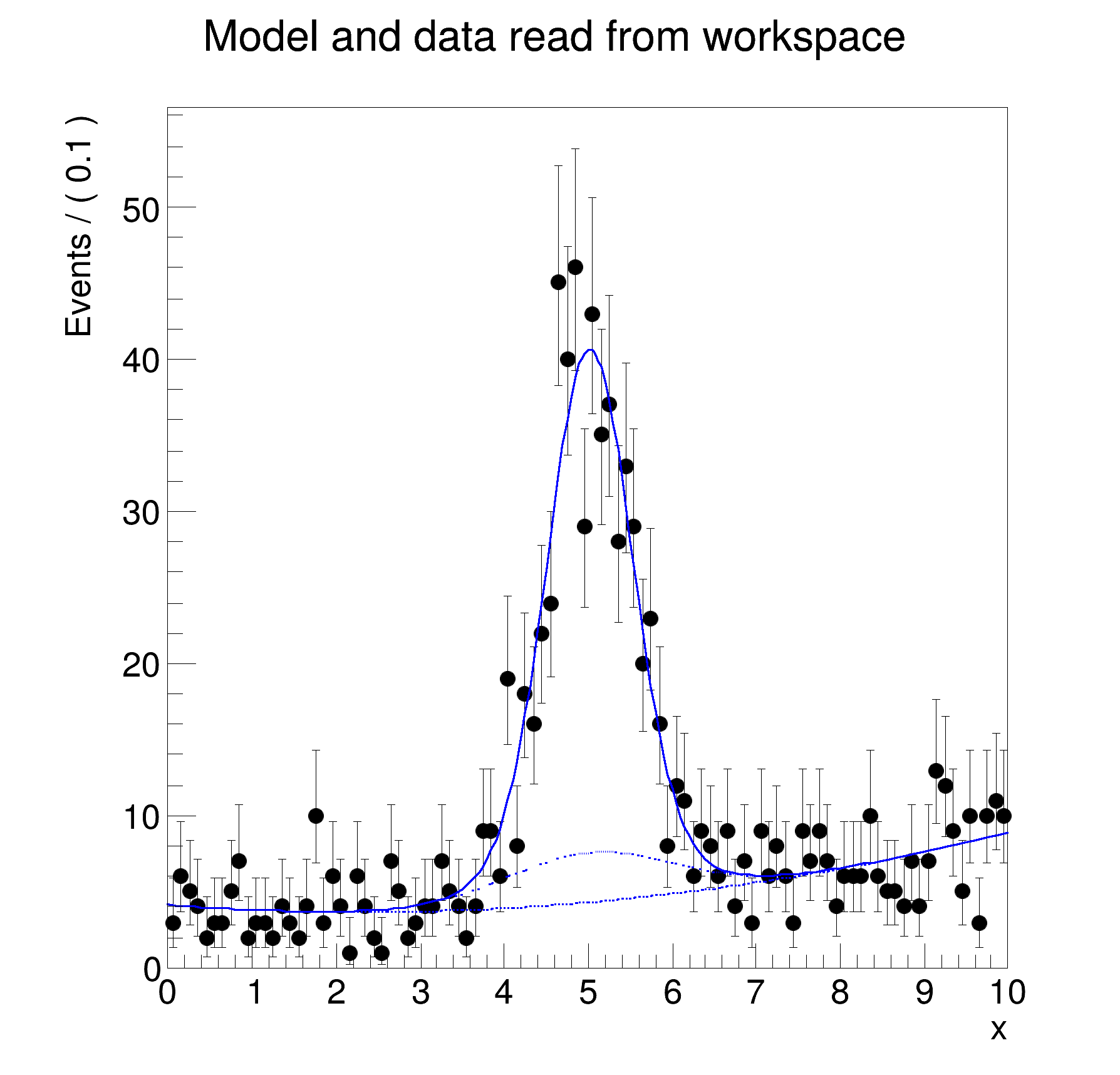ROOT   Reference Guide
Searching...
No Matches

## Detailed DescriptionOrganisation and simultaneous fits: reading and using a workspace

The input file for this macro is generated by rf502_wspacewrite.C#include "RooRealVar.h"
#include "RooDataSet.h"
#include "RooGaussian.h"
#include "RooChebychev.h"
#include "RooWorkspace.h"
#include "RooPlot.h"
#include "TCanvas.h"
#include "TAxis.h"
#include "TFile.h"
#include "TH1.h"
using namespace RooFit;
{
// R e a d w o r k s p a c e f r o m f i l e
// -----------------------------------------------
// Open input file with workspace (generated by rf503_wspacewrite)
TFile *f = new TFile("rf502_workspace.root");
// Retrieve workspace from file
RooWorkspace *w = (RooWorkspace *)f->Get("w");
// R e t r i e v e p d f , d a t a f r o m w o r k s p a c e
// -----------------------------------------------------------------
// Retrieve x,model and data from workspace
RooRealVar *x = w->var("x");
RooAbsPdf *model = w->pdf("model");
RooAbsData *data = w->data("modelData");
// Print structure of composite pdf
model->Print("t");
// F i t m o d e l t o d a t a , p l o t m o d e l
// ---------------------------------------------------------
// Fit model to data
model->fitTo(*data, PrintLevel(-1));
// Plot data and PDF overlaid
RooPlot *xframe = x->frame(Title("Model and data read from workspace"));
data->plotOn(xframe);
model->plotOn(xframe);
// Overlay the background component of model with a dashed line
model->plotOn(xframe, Components("bkg"), LineStyle(kDashed));
// Overlay the background+sig2 components of model with a dotted line
model->plotOn(xframe, Components("bkg,sig2"), LineStyle(kDotted));
// Draw the frame on the canvas
xframe->GetYaxis()->SetTitleOffset(1.4);
xframe->Draw();
}
#define f(i)
Definition RSha256.hxx:104
@ kDashed
Definition TAttLine.h:48
@ kDotted
Definition TAttLine.h:48
Option_t Option_t TPoint TPoint const char GetTextMagnitude GetFillStyle GetLineColor GetLineWidth GetMarkerStyle GetTextAlign GetTextColor GetTextSize void data
RooAbsData is the common abstract base class for binned and unbinned datasets.
Definition RooAbsData.h:57
A RooPlot is a plot frame and a container for graphics objects within that frame.
Definition RooPlot.h:43
static RooPlot * frame(const RooAbsRealLValue &var, double xmin, double xmax, Int_t nBins)
Create a new frame for a given variable in x.
Definition RooPlot.cxx:239
TAxis * GetYaxis() const
Definition RooPlot.cxx:1279
void Draw(Option_t *options=nullptr) override
Draw this plot and all of the elements it contains.
Definition RooPlot.cxx:652
RooRealVar represents a variable that can be changed from the outside.
Definition RooRealVar.h:37
The RooWorkspace is a persistable container for RooFit projects.
virtual void SetTitleOffset(Float_t offset=1)
Set distance between the axis and the axis title.
Definition TAttAxis.cxx:298
The Canvas class.
Definition TCanvas.h:23
A ROOT file is composed of a header, followed by consecutive data records (TKey instances) with a wel...
Definition TFile.h:54
Double_t x[n]
Definition legend1.C:17
The namespace RooFit contains mostly switches that change the behaviour of functions of PDFs (or othe...
0x557873f46310/V- RooChebychev::bkg = 0.8 [Auto,Dirty]
0x557873b174d0/V- RooRealVar::x = 5
0x557874019b90/V- RooRealVar::a0 = 0.5
0x55787401a390/V- RooRealVar::a1 = 0.2
0x557873f944f0/V- RooGaussian::sig1 = 1 [Auto,Dirty]
0x557873b174d0/V- RooRealVar::x = 5
0x557871d33910/V- RooRealVar::mean = 5
0x557874018e50/V- RooRealVar::sigma1 = 0.5
0x557871ccf2c0/V- RooRealVar::sig1frac = 0.8
0x557873fbd150/V- RooGaussian::sig2 = 1 [Auto,Dirty]
0x557873b174d0/V- RooRealVar::x = 5
0x557871d33910/V- RooRealVar::mean = 5
0x557872334320/V- RooRealVar::sigma2 = 1
[#1] INFO:Minimization -- RooAbsMinimizerFcn::setOptimizeConst: activating const optimization
[#1] INFO:Minimization -- The following expressions will be evaluated in cache-and-track mode: (bkg,sig1,sig2)
[#1] INFO:Minimization -- RooAbsMinimizerFcn::setOptimizeConst: deactivating const optimization
[#1] INFO:Plotting -- RooAbsPdf::plotOn(model) directly selected PDF components: (bkg)
[#1] INFO:Plotting -- RooAbsPdf::plotOn(model) indirectly selected PDF components: ()
[#1] INFO:Plotting -- RooAbsPdf::plotOn(model) directly selected PDF components: (bkg,sig2)
[#1] INFO:Plotting -- RooAbsPdf::plotOn(model) indirectly selected PDF components: (sig)
Date
July 2008